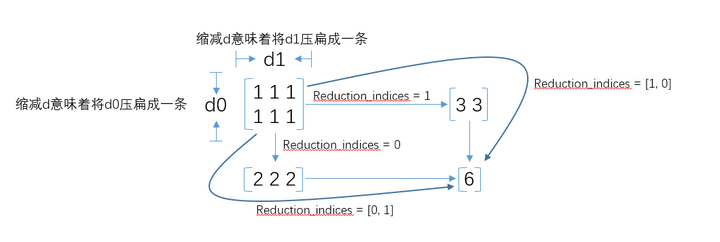## [TensorFlow]Tensor维度理解_tensorflow tensor的维度怎么看_hangkai_tan的博客-程序员秘密

http://wossoneri.github.io/2017/11/15/[Tensorflow]The-dimension-of-Tensor/

### Tensor维度理解

Tensor在Tensorflow中是N维矩阵，所以涉及到Tensor的方法，也都是对矩阵的处理。由于是多维，在Tensorflow中Tensor的流动过程就涉及到升维降维，这篇就通过一些接口的使用，来体会Tensor的维度概念。以下是个人体会，有不准确的请指出。

tf.reduce_mean

``````reduce_mean(
input_tensor,
axis=None,
keep_dims=False,
name=None,
reduction_indices=None
)``````

``````x = tf.constant([[1., 1.], [2., 2.]])
tf.reduce_mean(x)  # 1.5
tf.reduce_mean(x, 0)  # [1.5, 1.5]
tf.reduce_mean(x, 1)  # [1.,  2.]``````

x是二维数组[[1.0,1.0],[2.0, 2.0]]
`axis`参数取默认值时，计算整个数组的均值：(1.+1.+2.+2.)/4=1.5
`axis`取0，意味着对列取均值：[1.5, 1.5]
`axis`取1，意味着对行取均值：[1.0, 2.0]

``````sess = tf.Session()
x = tf.constant([[1., 2., 3.], [4., 5., 6.], [7., 8., 9.]])
print(sess.run(x))
print(sess.run(tf.reduce_mean(x)))
print(sess.run(tf.reduce_mean(x, 0)))
print(sess.run(tf.reduce_mean(x, 1)))``````

``````[[ 1.  2.  3.]
[ 4.  5.  6.]
[ 7.  8.  9.]]
5.0
[ 4.  5.  6.]
[ 2.  5.  8.]``````

``````sess = tf.Session()
x = tf.constant([[[1., 1.], [2., 2.]], [[3., 3.], [4., 4.]]])
print(sess.run(x))
print(sess.run(tf.reduce_mean(x)))
print(sess.run(tf.reduce_mean(x, 0)))
print(sess.run(tf.reduce_mean(x, 1)))
print(sess.run(tf.reduce_mean(x, 2)))``````

``````[[[ 1.  1.]
[ 2.  2.]]

[[ 3.  3.]
[ 4.  4.]]]``````

``````2.5
[[ 2.  2.]
[ 3.  3.]]
[[ 1.5  1.5]
[ 3.5  3.5]]
[[ 1.  2.]
[ 3.  4.]]``````

`axis`参数取默认值时，依然计算整个数组的均值：(float)(1+2+3+4+1+2+3+4)/8=2.5
`axis`取0，计算方式是：

``````[[(1+3)/2, (1+3)/2],
[(2+4)/2, (2+4)/2]]``````

`axis`取1，计算方式是：

``````[[(1+2)/2, (1+2)/2],
[(3+4)/2, (3+4)/2]]``````

`axis`取2，计算方式是：

``````[[(1+1)/2, (2+2)/2],
[(3+3)/2, (4+4)/2]]``````

tf.reduce_xyz(x, axis=k-1)的结果是对最里面一维所有元素进行求和。
tf.reduce_xyz(x, axis=k-2)是对倒数第二层里的向量对应的元素进行求和。
tf.reduce_xyz(x, axis=k-3)把倒数第三层的每个向量对应元素相加。

``````[[[ 1.  1.]
[ 2.  2.]]

[[ 3.  3.]
[ 4.  4.]]]``````

axis=3-1=2，做最内层计算，我们的最内层就是(1,1),(2,2),(3,3),(4,4)，计算出来的就是

``````[[ 1.  2.]
[ 3.  4.]]``````

axis=3-2=1，做倒数第二层计算(参考二维计算)：([1,1],[2,2])和([3, 3],[4, 4])

``````[[ 1.5  1.5]
[ 3.5  3.5]]``````

axis=3-3=1，做倒数第三层计算:([[1, 1], [2, 2]])([[3, 3], [4, 4]])

``````[[ 2.  2.]
[ 3.  3.]]``````

``````# input 4-D
[[[[ 1.  1.]
[ 2.  2.]]

[[ 3.  3.]
[ 4.  4.]]]

[[[ 5.  5.]
[ 6.  6.]]

[[ 7.  7.]
[ 8.  8.]]]]
# axis=none
4.5

# axis=0
[[[ 3.  3.]
[ 4.  4.]]

[[ 5.  5.]
[ 6.  6.]]]

# axis=1
[[[ 2.  2.]
[ 3.  3.]]

[[ 6.  6.]
[ 7.  7.]]]``````

the rank of the tensor is reduced by 1 for each entry in axis``````# 2*2
[[ 1.  1.]
[ 2.  2.]]
# keep_dims=False
[ 1.5  1.5] # 1*2
[ 1.  2.]   #1*2
# keep_dims=True
[[ 1.5  1.5]]   #1*2
[[ 1.]          #2*1
[ 2.]]``````

### zbrush-拓扑低模_zb高模怎么拓扑成低模_蝶泳奈何桥.的博客-程序员秘密

title: art-zbrush-zsphere_topology_拓扑低模categories: Arttags: [art, zbrush, ta, topology, 拓扑]date: 2019-01-03 17:03:18comments: falseart-zbrush-zsphere_topology_拓扑低模zshpere 拓扑低模拓扑模型 topology...

### python堆栈跟踪_traceback (Runtime) – Python 中文开发手册 - Break易站_weixin_39552317的博客-程序员秘密

Python 中文开发手册traceback (Runtime) - Python 中文开发手册该模块提供了一个标准接口来提取，格式化和打印Python程序的堆栈跟踪。它完全模仿Python解释器在打印堆栈跟踪时的行为。当您想要在程序控制下打印堆栈跟踪时，例如在解释器周围的“包装器”中，这很有用。模块使用跟踪对象 - 这是存储在变量sys.exc_traceback（不建议使用）和sys.last...

### MacOS BigSur 在根目录下生成/data_绿叶上的露珠的博客-程序员秘密

MacOS BigSur 在根目录下生成/data最近刚换了Mac 由于项目中需要在/data/logs打印日志，但是发现MacOS Big Sur无法在根目录下创建data文件夹，查阅资料加以实践后终于得到解决，现分享给大家。解决方法如下：1、进入/System/Volumes/Data并创建data文件夹cd /System/Volumes/Datasudo mkdir datasudo chmod -R 777 /data2、打开synthetic.conf（没有就新建一个）并写入以下

### MyEclipse设置代码提示功能_myeclipse提示框文字_知也无涯的博客-程序员秘密

package test;import javax.swing.*;import javax.swing.border.EmptyBorder;import javax.swing.filechooser.FileSystemView;import java.awt.*;import java.awt.event.ActionEvent;import java.awt.event....

### 如何使用SetTimer MFC_ellor的博客-程序员秘密

Timer事件，即定时器事件，是在游戏编程中，经常使用的一个事件。借助它可以产生定时执行动作的效果。这篇文章，就和大家一起探讨一下如何使用SetTimer（）函数。           1、SetTimer定义在那里？            SetTimer表示的是定义个定时器。根据定义指定的窗口，在指定的窗口（CWnd）中实现OnTimer事件，这样，就可以相应事件了。SetTi

### 配置OpenSSL时候报nmake不是内部命令或外部命令解决方法_befreedomjustice的博客-程序员秘密

cmd到vc目录下，运行vcvarsall.bat，然后再cd到openssl目录nmake剪切 (Ctrl+X)复制 (Ctrl+C)粘贴 (Ctrl+V)粘贴文本段落标签字体字体大小加粗 (Ctrl+B)斜体 (Ctrl+I)下划线 (Ctrl+U)删除线字体颜色背景颜色删除文字格式对齐列表减少缩进增加缩进超链接 (Ctrl+L)取消超链接图